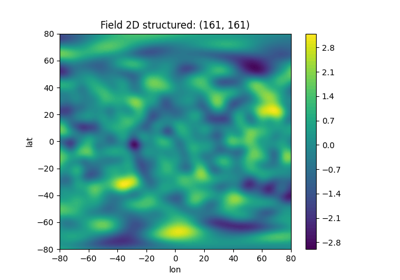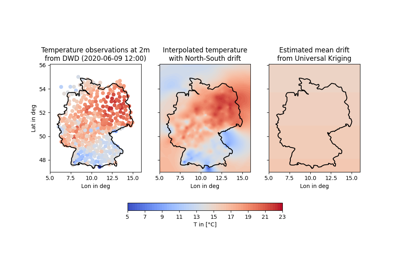# Geographic Coordinates¶

GSTools provides support for geographic coordinates given by:

• latitude lat: specifies the north–south position of a point on the Earth’s surface

• longitude lon: specifies the east–west position of a point on the Earth’s surface

If you want to use this feature for field generation or Kriging, you have to set up a geographical covariance Model by setting latlon=True in your desired model (see CovModel):

import numpy as np
import gstools as gs

model = gs.Gaussian(latlon=True, var=2, len_scale=np.pi / 16)


By doing so, the model will use the associated Yadrenko model on a sphere (see here). The len_scale is given in radians to scale the arc-length. In order to have a more meaningful length scale, one can use the rescale argument:

import gstools as gs

model = gs.Gaussian(latlon=True, var=2, len_scale=500, rescale=gs.EARTH_RADIUS)


Then len_scale can be interpreted as given in km.

A Yadrenko modelis derived from a valid isotropic covariance model in 3Dby the following relation:Whereis the great-circle distance.

Note

lat and lon are given in degree, whereas the great-circle distanceis given in radians.

Note, thatis the chordal distance of two points on a sphere, which means we simply think of the earth surface as a sphere, that is cut out of the surrounding three dimensional space, when using the Yadrenko model.

Note

Anisotropy is not available with the geographical models, since their geometry is not euclidean. When passing values for CovModel.anis or CovModel.angles, they will be ignored.

Since the Yadrenko model comes from a 3D model, the model dimension will be 3 (see CovModel.dim) but the field_dim will be 2 in this case (see CovModel.field_dim).

## Examples¶Gallery generated by Sphinx-Gallery